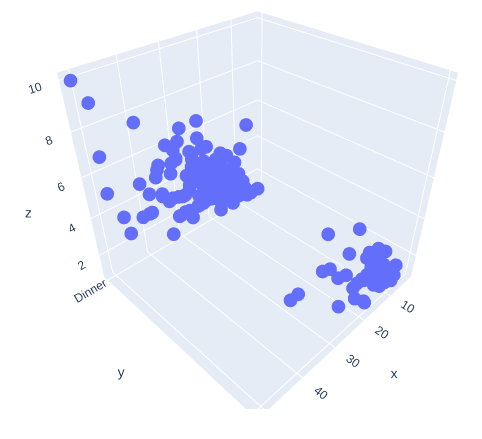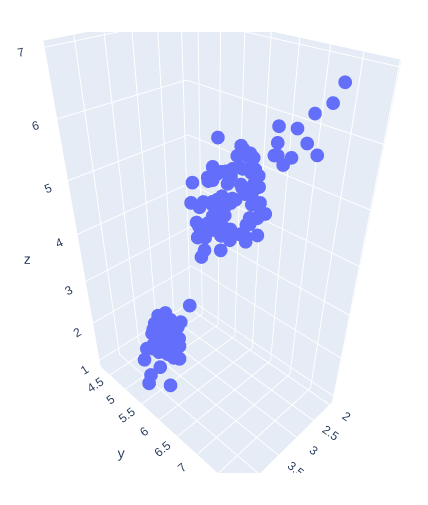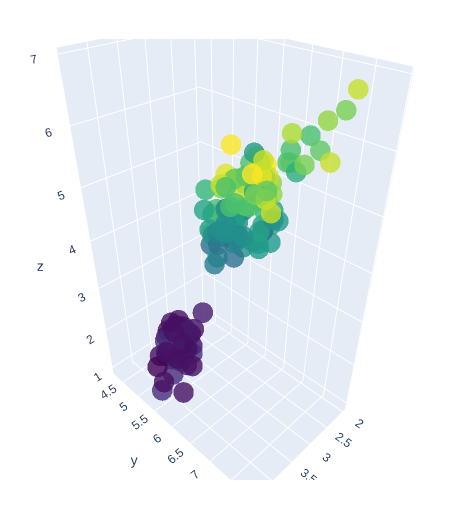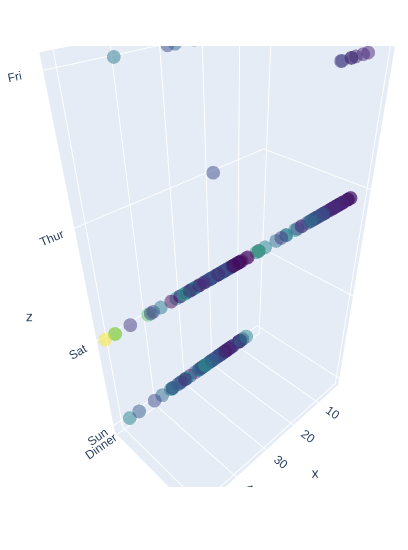# 3D Scatter Plot using graph_objects Class in Plotly-Python

Plotly is a Python library that is used to design graphs, especially interactive graphs. It can plot various graphs and charts like histogram, barplot, boxplot, spreadplot, and many more. It is mainly used in data analysis as well as financial analysis. plotly is an interactive visualization library.

## Scatter plot using graph_objects class

If a plotly express does not provide a good starting point, then it is possible to use go.Scatter3D class from plotly.graph_objects. Scatter plot are those charts in which data points are represented horizontally and on the vertical axis to show how one variable affect on another variable. The mode of the property decides the appearance of data points.

Syntax: plotly.graph_objects.Scatter3d(arg=None, connectgaps=None, customdata=None, customdatasrc=None, error_x=None, error_y=None, error_z=None, hoverinfo=None, hoverinfosrc=None, hoverlabel=None, hovertemplate=None, hovertemplatesrc=None, hovertext=None, hovertextsrc=None, ids=None, idssrc=None, legendgroup=None, line=None, marker=None, meta=None, metasrc=None, mode=None, name=None, opacity=None, projection=None, scene=None, showlegend=None, stream=None, surfaceaxis=None, surfacecolor=None, text=None, textfont=None, textposition=None, textpositionsrc=None, textsrc=None, texttemplate=None, texttemplatesrc=None, uid=None, uirevision=None, visible=None, x=None, xcalendar=None, xsrc=None, y=None, ycalendar=None, ysrc=None, z=None, zcalendar=None, zsrc=None, **kwargs)

Parameters:

x – Sets the x coordinates.

y – Sets the y coordinates.

z – Sets the z coordinates.

mode – Determines the drawing mode for this scatter trace.

Example 1:

## Python3

 `import` `plotly.express as px ` `import` `plotly.graph_objects as go ` ` `  `df ``=` `px.data.tips() ` ` `  `fig ``=` `go.Figure(data ``=``[go.Scatter3d(x ``=` `df[``'total_bill'``], ` `                                   ``y ``=` `df[``'time'``], ` `                                   ``z ``=` `df[``'tip'``], ` `                                   ``mode ``=``'markers'``)]) ` `fig.show()`

Output:Example 2:

## Python3

 `import` `plotly.express as px ` `import` `plotly.graph_objects as go ` ` `  `df ``=` `px.data.iris() ` ` `  `fig ``=` `go.Figure(data ``=``[go.Scatter3d(x ``=` `df[``'sepal_width'``], ` `                                   ``y ``=` `df[``'sepal_length'``], ` `                                   ``z ``=` `df[``'petal_length'``], ` `                                   ``mode ``=``'markers'``)]) ` `fig.show()`

Output:## Presenting 3D Scatter Plot with Color scaling and Marker Styling

In plotly, color scaling and marker styling are a way to represent the data more effectively, and it makes data more understandable.

Example 1:

## Python3

 `import` `plotly.express as px ` `import` `plotly.graph_objects as go ` ` `  `df ``=` `px.data.iris() ` ` `  `fig ``=` `go.Figure(data ``=``[go.Scatter3d(x ``=` `df[``'sepal_width'``], ` `                                   ``y ``=` `df[``'sepal_length'``], ` `                                   ``z ``=` `df[``'petal_length'``], ` `                                   ``mode ``=``'markers'``,  ` `                                   ``marker ``=` `dict``( ` `                                     ``size ``=` `12``, ` `                                     ``color ``=` `df[``'petal_width'``], ` `                                     ``colorscale ``=``'Viridis'``, ` `                                     ``opacity ``=` `0.8` `                                   ``) ` `)]) ` ` `  `fig.show()`

Output:Example 2:

## Python3

 `import` `plotly.express as px ` `import` `plotly.graph_objects as go ` ` `  `df ``=` `px.data.tips() ` ` `  `fig ``=` `go.Figure(data ``=``[go.Scatter3d(x ``=` `df[``'total_bill'``], ` `                                   ``y ``=` `df[``'time'``], ` `                                   ``z ``=` `df[``'day'``], ` `                                   ``mode ``=``'markers'``, ` `                                   ``marker ``=` `dict``( ` `                                     ``color ``=` `df[``'tip'``], ` `                                     ``colorscale ``=``'Viridis'``, ` `                                     ``opacity ``=` `0.5` `                                   ``) ` `)]) ` `fig.show()`

Output:Whether you're preparing for your first job interview or aiming to upskill in this ever-evolving tech landscape, GeeksforGeeks Courses are your key to success. We provide top-quality content at affordable prices, all geared towards accelerating your growth in a time-bound manner. Join the millions we've already empowered, and we're here to do the same for you. Don't miss out - check it out now!

Previous
Next